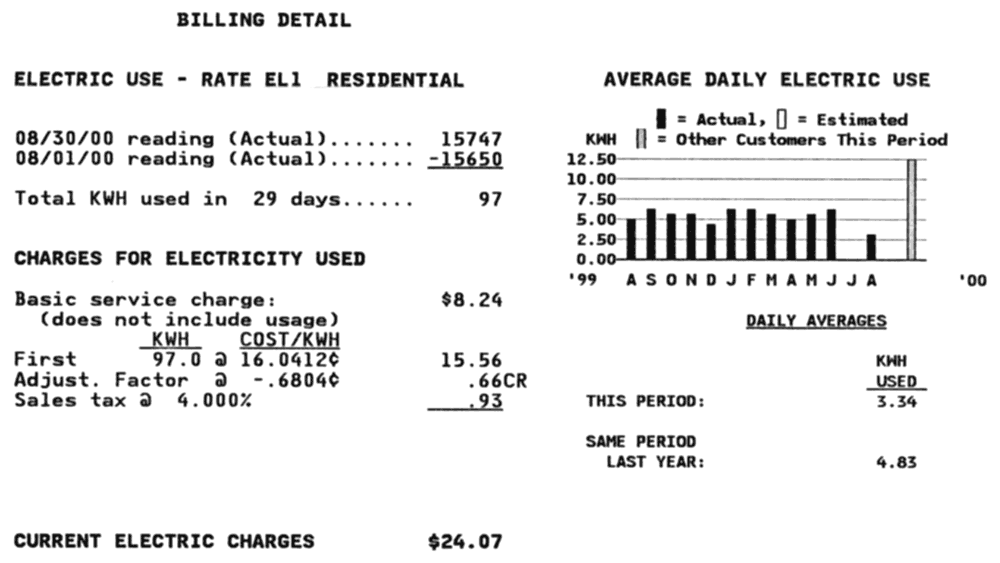The Physics
Hypertextbook
Opus in profectus

# Electric Power

## Discussion

James Joule (1818–1889) England, verified Ohm's Law and determined that the heat delivered by a conductor is directly proportional to its resistance and to the square of the current through it.

We see, therefore, that when a current of voltaic electricity is propagated along a metallic conductor, the heat evolved in a given time is proportional to the resistance of the conductor multiplied by the square of the electric intensity.

James Prescott Joule, 1841

Electric power from the definition of power. Multiply by one and commute the variables in the denominator. Look at what this gives us.

 P = W = W q = W q = VI t t q q t

This is the original definition of the watt as the unit of power.

The other unit I would suggest adding to the list is that of power. The power conveyed by a current of an ampère through the difference of potential of a volt is the unit consistent with the practical system. It might be appropriately called a watt, in honour of that master mind in mechanical science, James Watt. He it was who first had a clear physical conception of power, and gave a rational method of measuring it. A watt, then, expresses the rate of an ampère multiplied by a volt, whilst a horsepower is 746 watts, and a cheval de vapeur 735.

Carl Wilhelm Siemens, 1882

The horsepower is the unit for power invented by James Watt. The cheval de vapeur (literally the horse of steam) is the French name for what is often called the metric horsepower in English. Interestingly, the French call James Watt's horsepower le cheval-vapeur britannique.

Power in terms of current. Remove the voltage by substituting from Ohm's law.

P = VI = (IR)I = I2R

Power in terms of voltage. Remove the current using Ohm's law.

 P = VI = V V = V2 R R

In summary…

 P = VI = I2R = V2 R

Consumer affairs…A portion of a residential consumer electric bill. Utility companies sell electric energy by the kilowatt hour; a unit that simplifies calculating operating costs for electrical devices. The energy consumed during this particular billing cycle was quite small (when compared to similar consumers), but the rate charged by this utility was approximately double the US average in the year 2000.

Conventional (copper-based) cables are capable of transmitting power (40 to 600 MW) at high voltages (40 to 345 kV)

Magnify

A similar bill from 2015.

Line loss